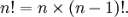## 关于阶乘的两个常见算法及一个相关面试题

2008-05-19 12:12 by Anders Cui, ... 阅读, ... 评论, 收藏, 编辑public static long Calculate(int n)
{
    if (n < 0) { throw new ArgumentOutOfRangeException("n必须为非负数。"); }
    if (n == 0) { return 1; }

    return n * Calculate(n - 1);
}

public static uint[] CalculateLargeNumber(int n)
{
    if (n < 0) { throw new ArgumentOutOfRangeException("n必须为非负数。"); }
    if (n == 0 || n == 1) { return new uint[] { 1 }; }

    // 数组的最大长度
    const int MaxLength = 100000;
    uint[] array = new uint[MaxLength];
    // 1! = 1
    array = 1;

    int i = 0;
    int j = 0;
    // valid为当前阶乘值的位数（如5! = 120，此时valid = 3）
    int valid = 1;
    for (i = 2; i <= n; i++)
    {
        long carry = 0;
        for (j = 0; j < valid; j++)
        {
            long multipleResult = array[j] * i + carry;
            // 计算当前位的数值
            array[j] = (uint)(multipleResult % 10);
            // 计算进到高位的数值
            carry = multipleResult / 10;
        }
        // 为更高位赋值
        while (carry != 0)
        {
            array[valid++] = (uint)(carry % 10);
            carry /= 10;
        }
    }

    // 截取有效元素
    uint[] result = new uint[valid];
    Array.Copy(array, result, valid);

    return result;
}

http://en.wikipedia.org/wiki/Factorial